# RD Sharma Solutions For Class 7 Maths Chapter 15 Properties of Triangles Exercise 15.3

Students can view and download the PDF of RD Sharma Solutions for Class 7 Maths Exercise 15.3 of Chapter 15 Properties of Triangles available here. The BYJU’S experts in Maths formulate the solutions for questions present in the textbook based on the understanding abilities of students. This exercise explains exterior and interior angles of a triangle. RD Sharma Solutions for Class 7 includes answers to all questions present in this exercise. Several topics of this exercise are listed below:

• Exterior angle
• Interior opposite angle
• Theorem based on the adjacent angle

## Download the PDF of RD Sharma Solutions For Class 7 Maths Chapter 15 – Properties of Triangles Exercise 15.3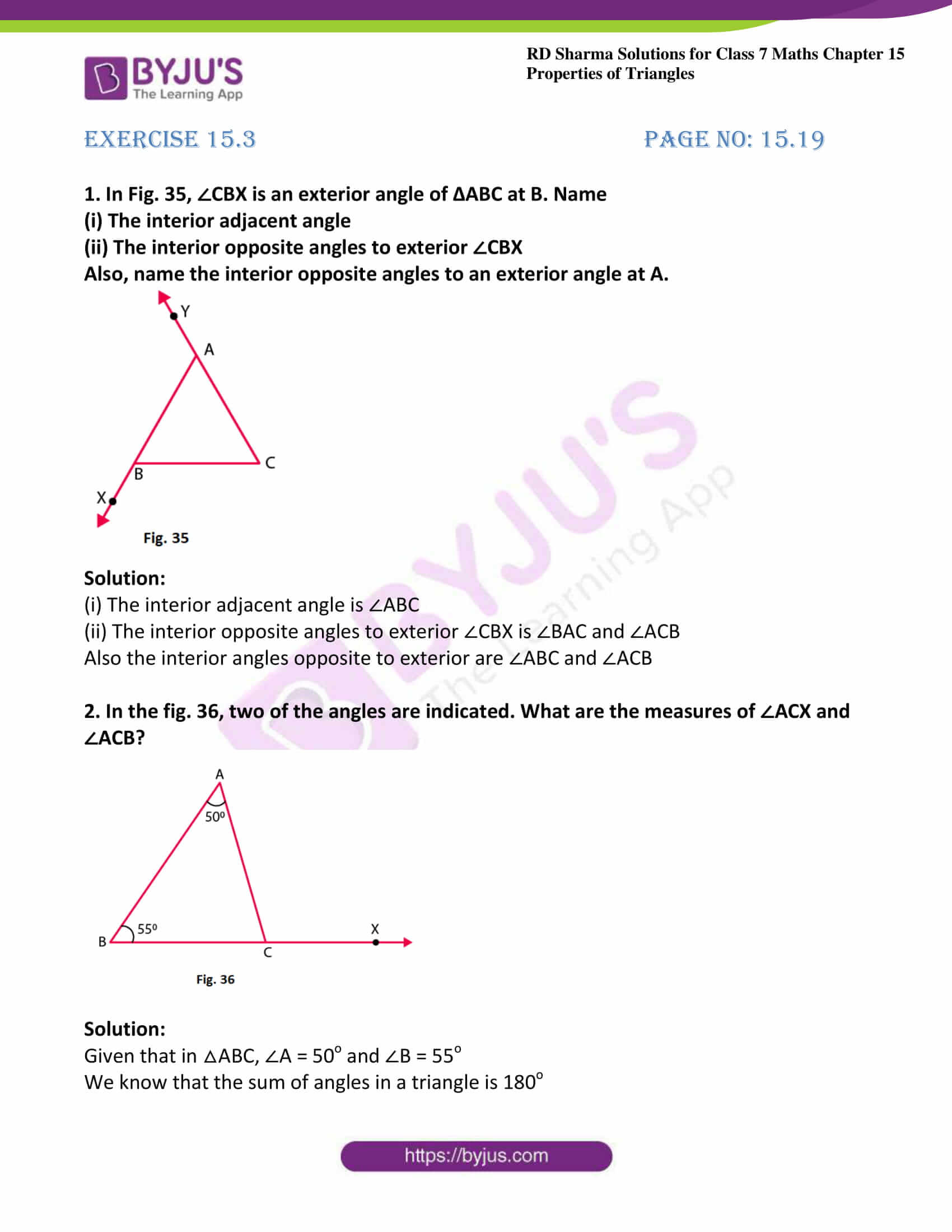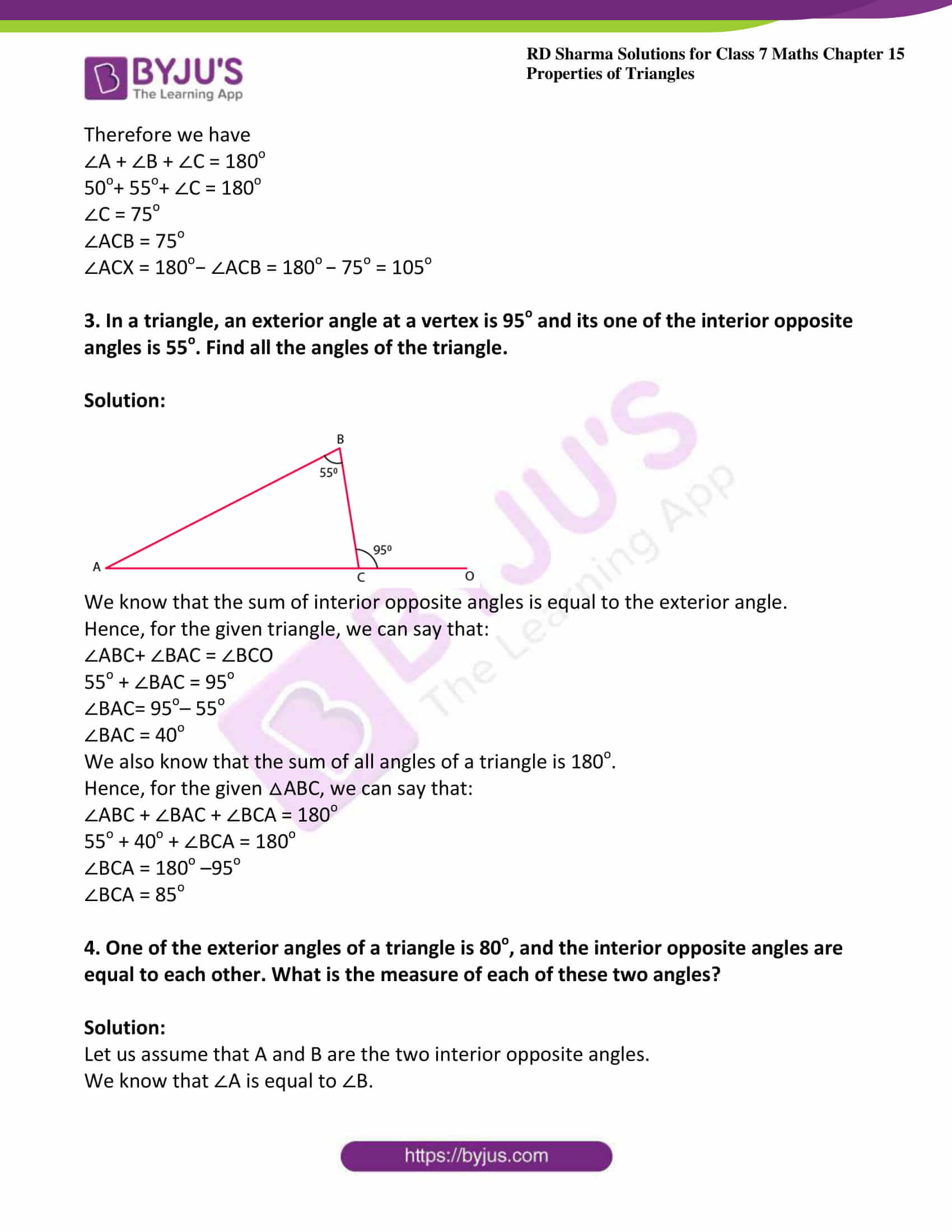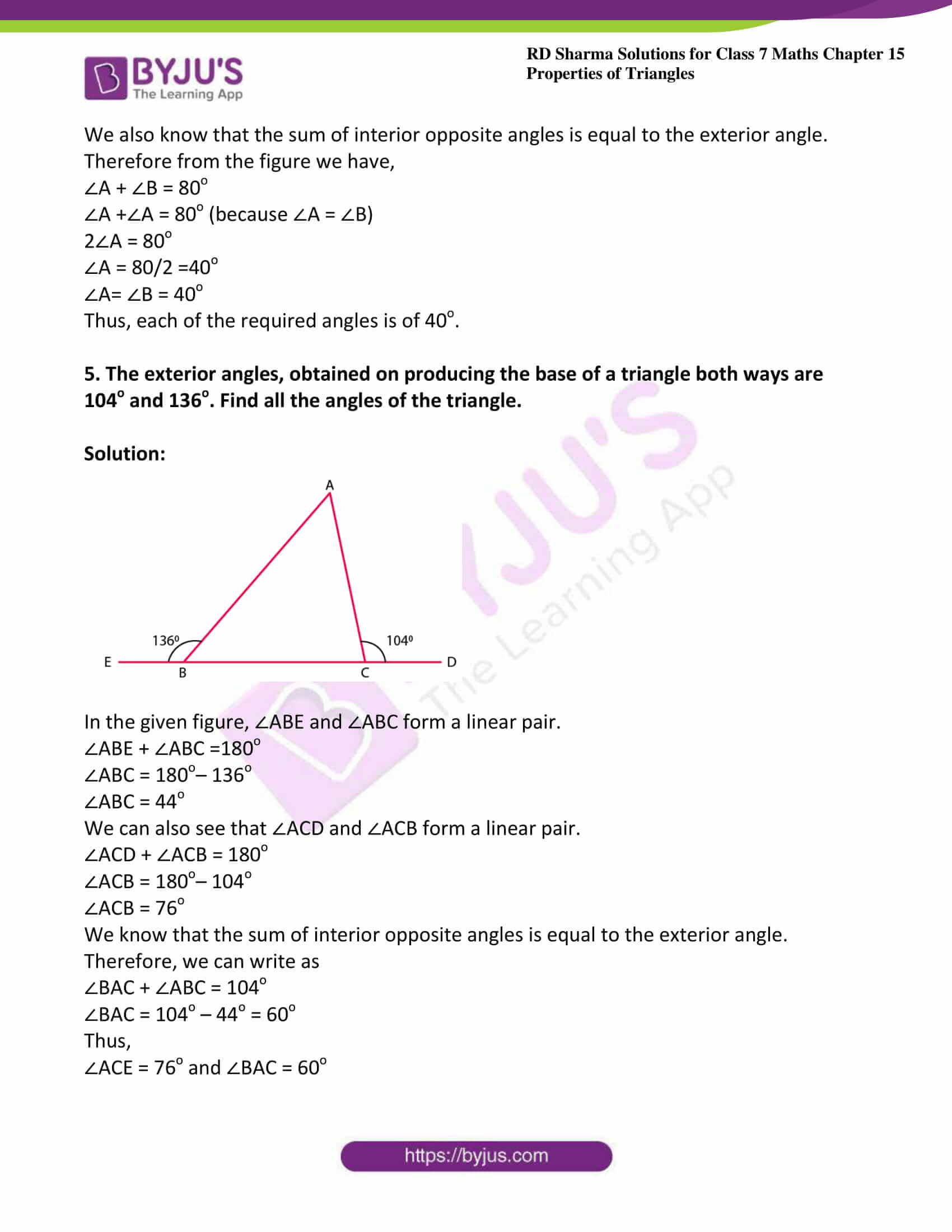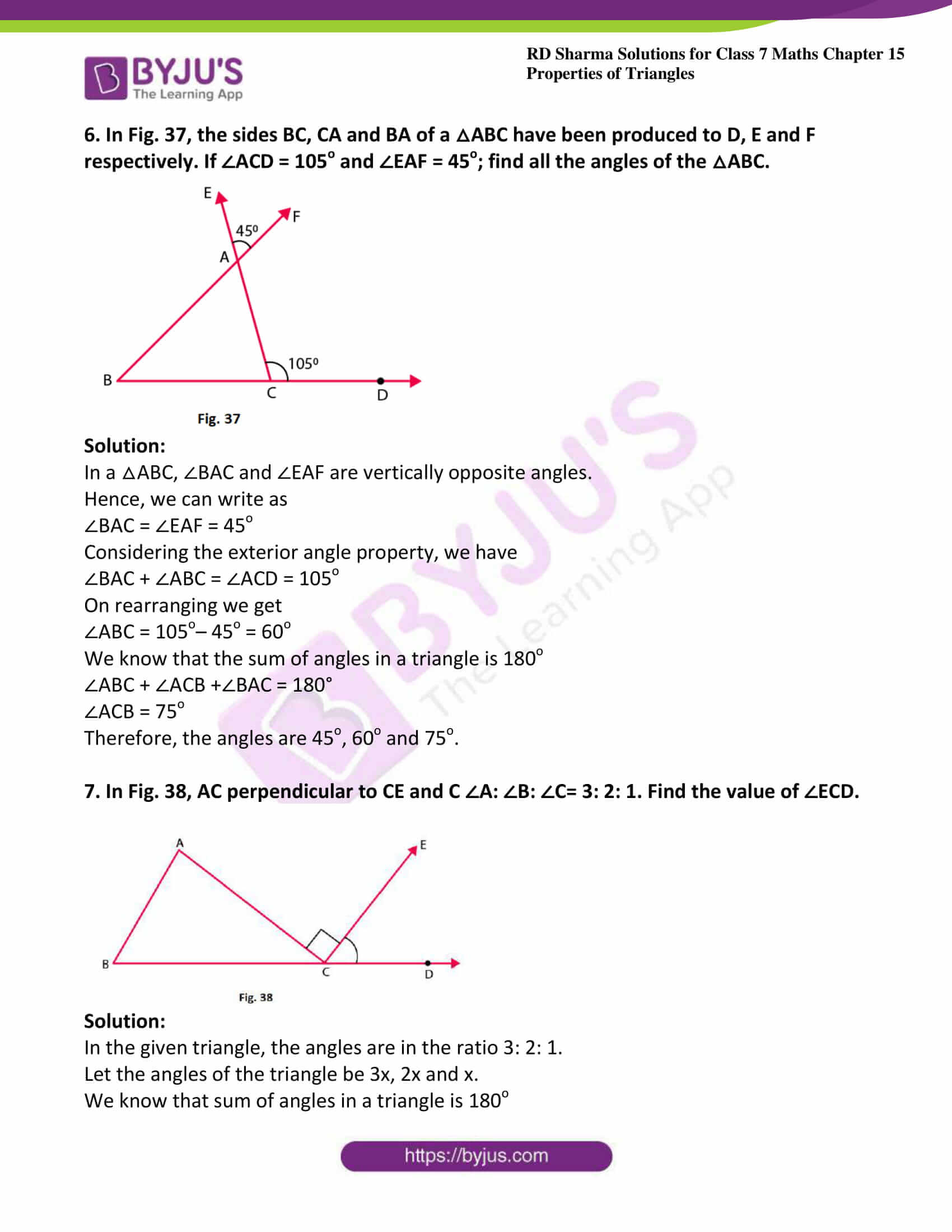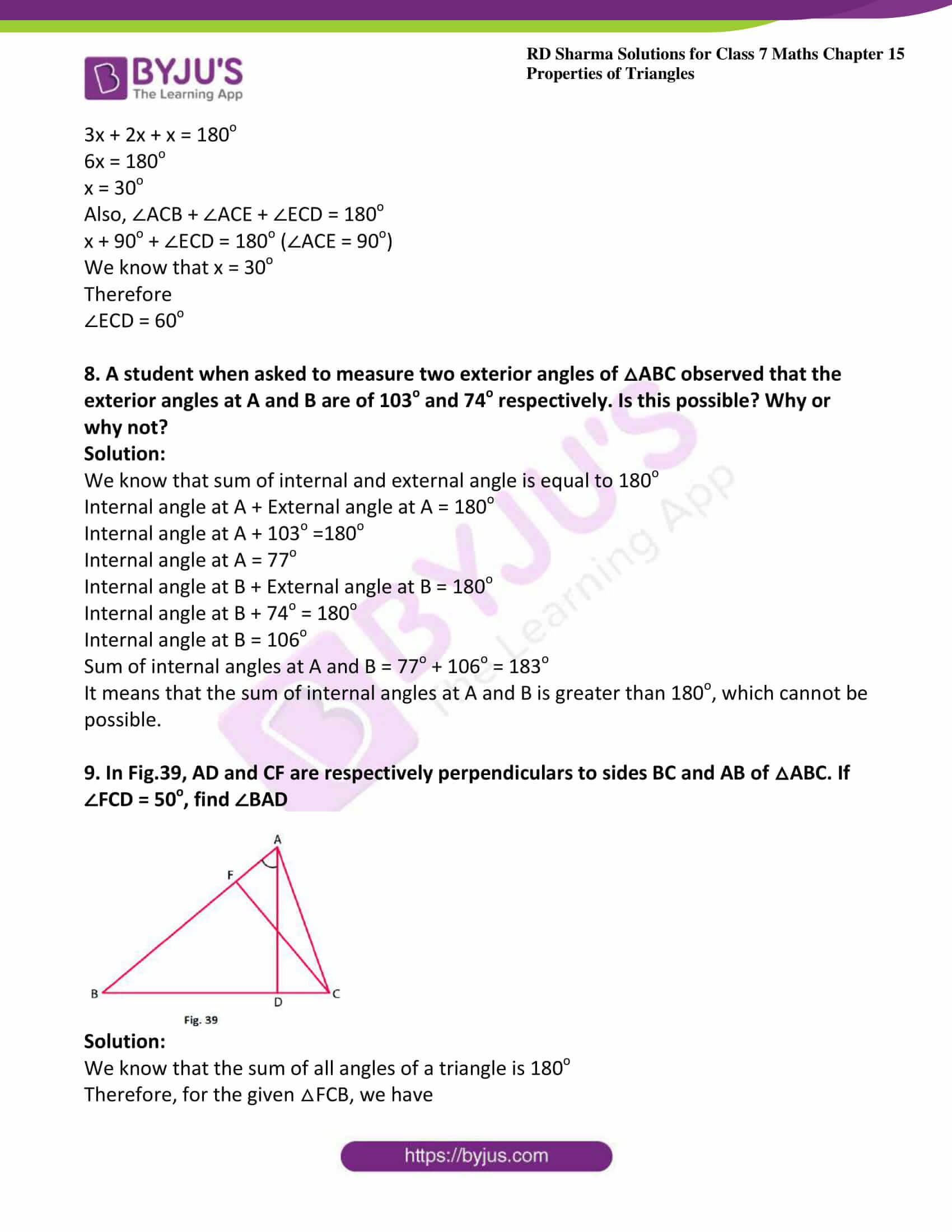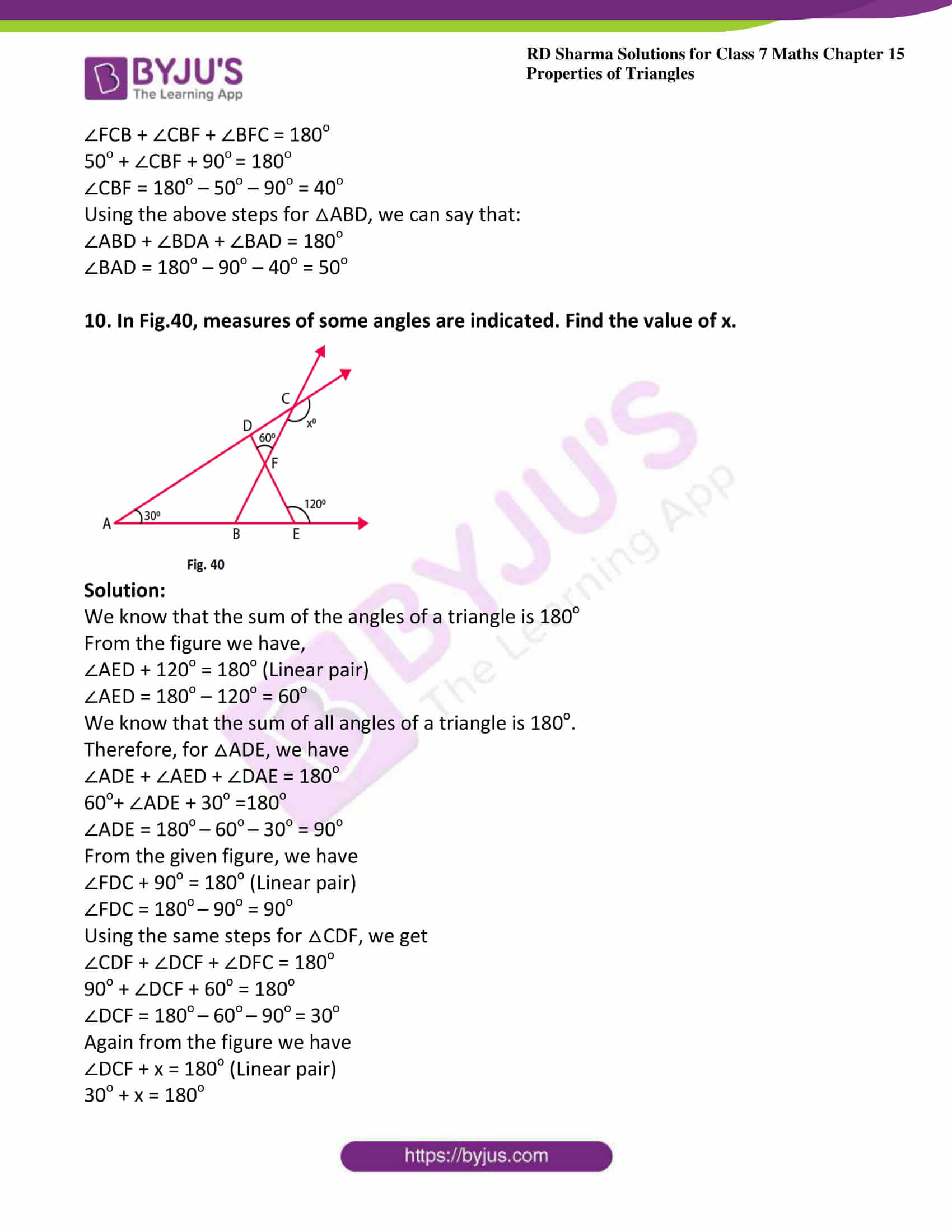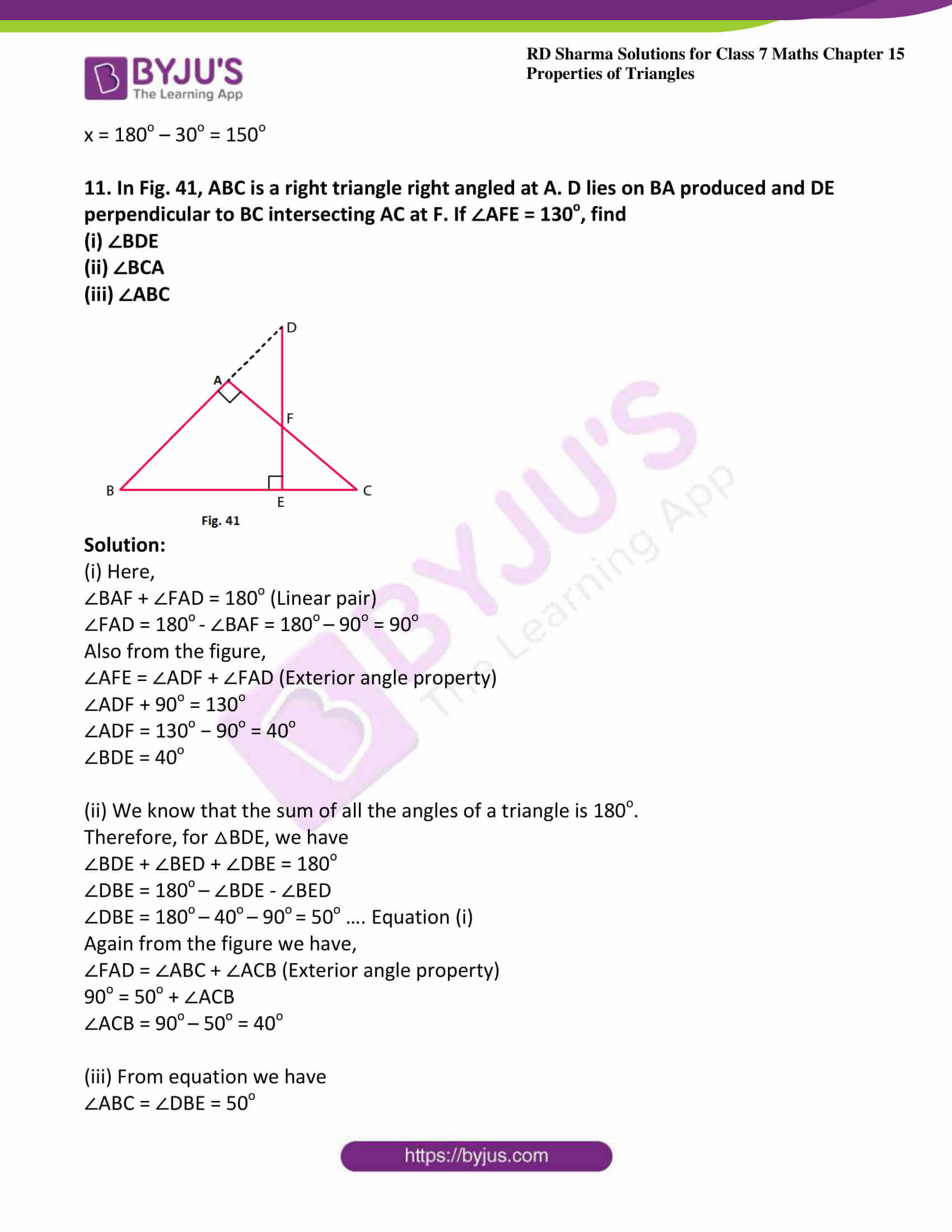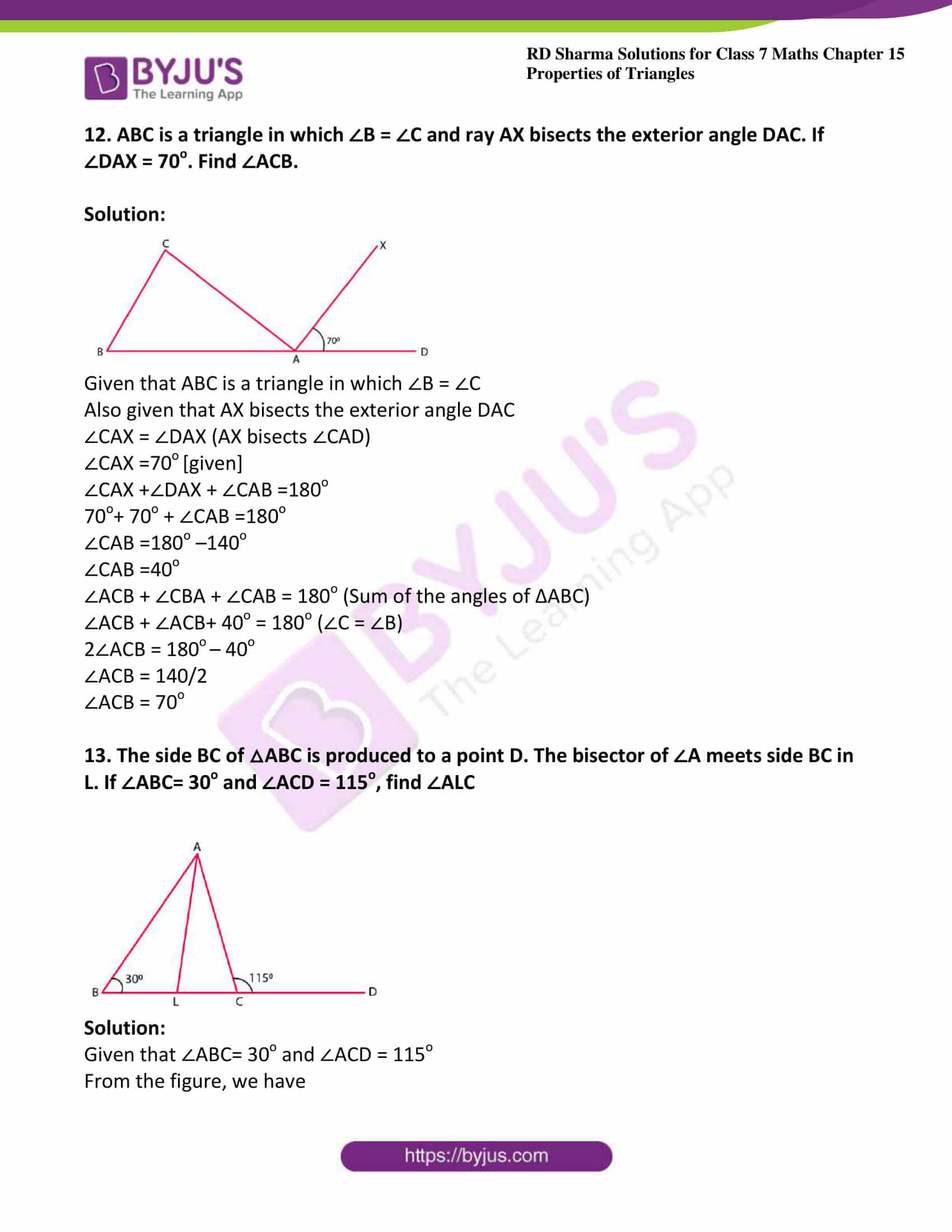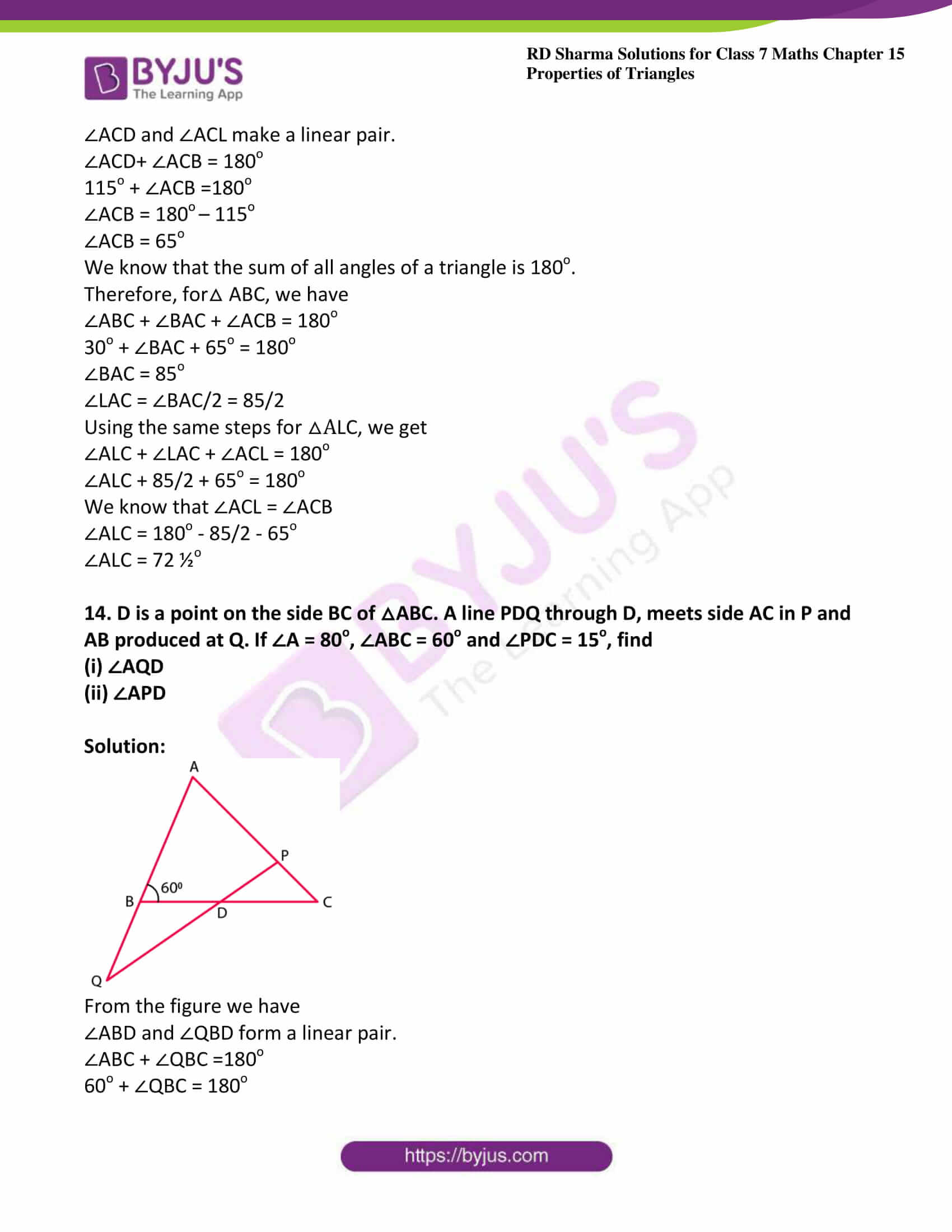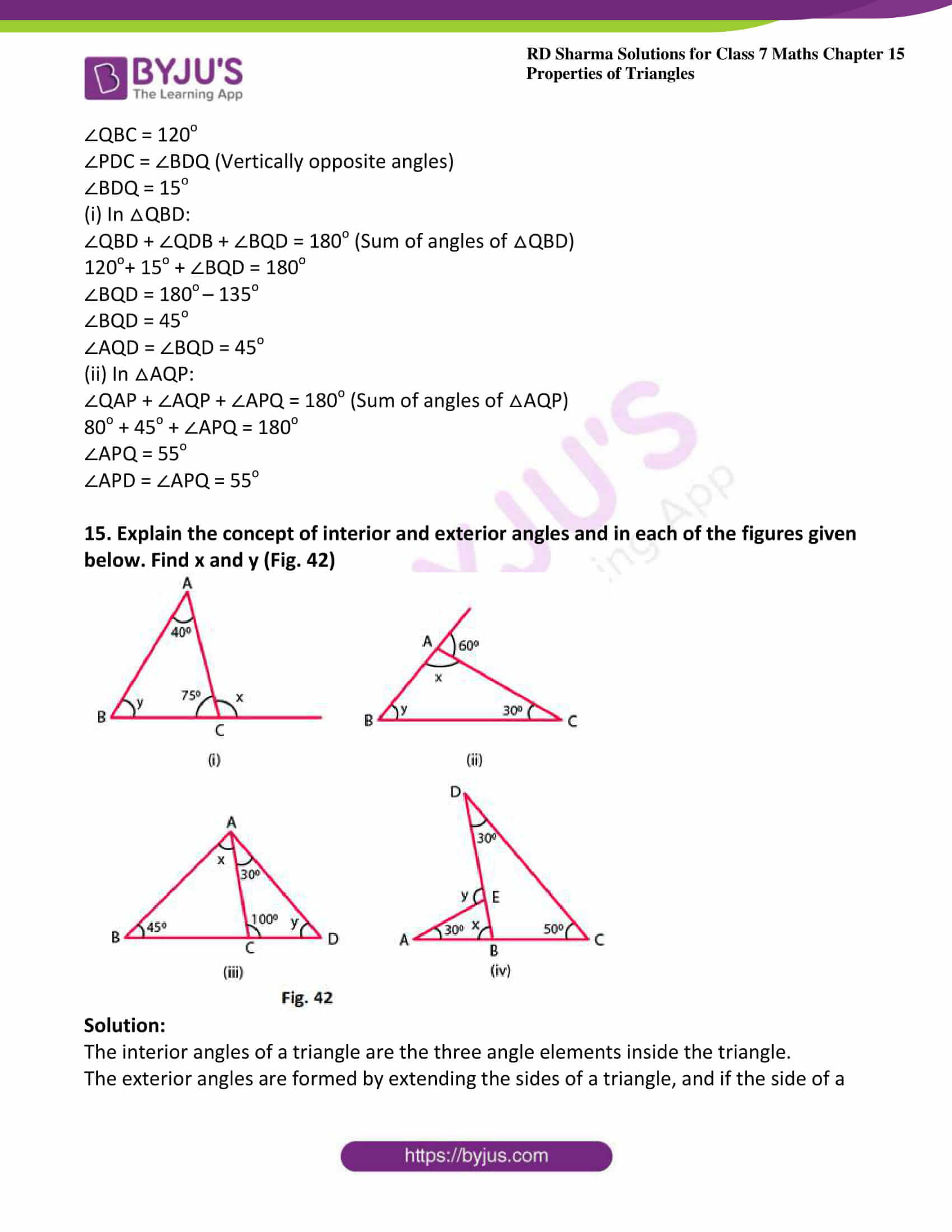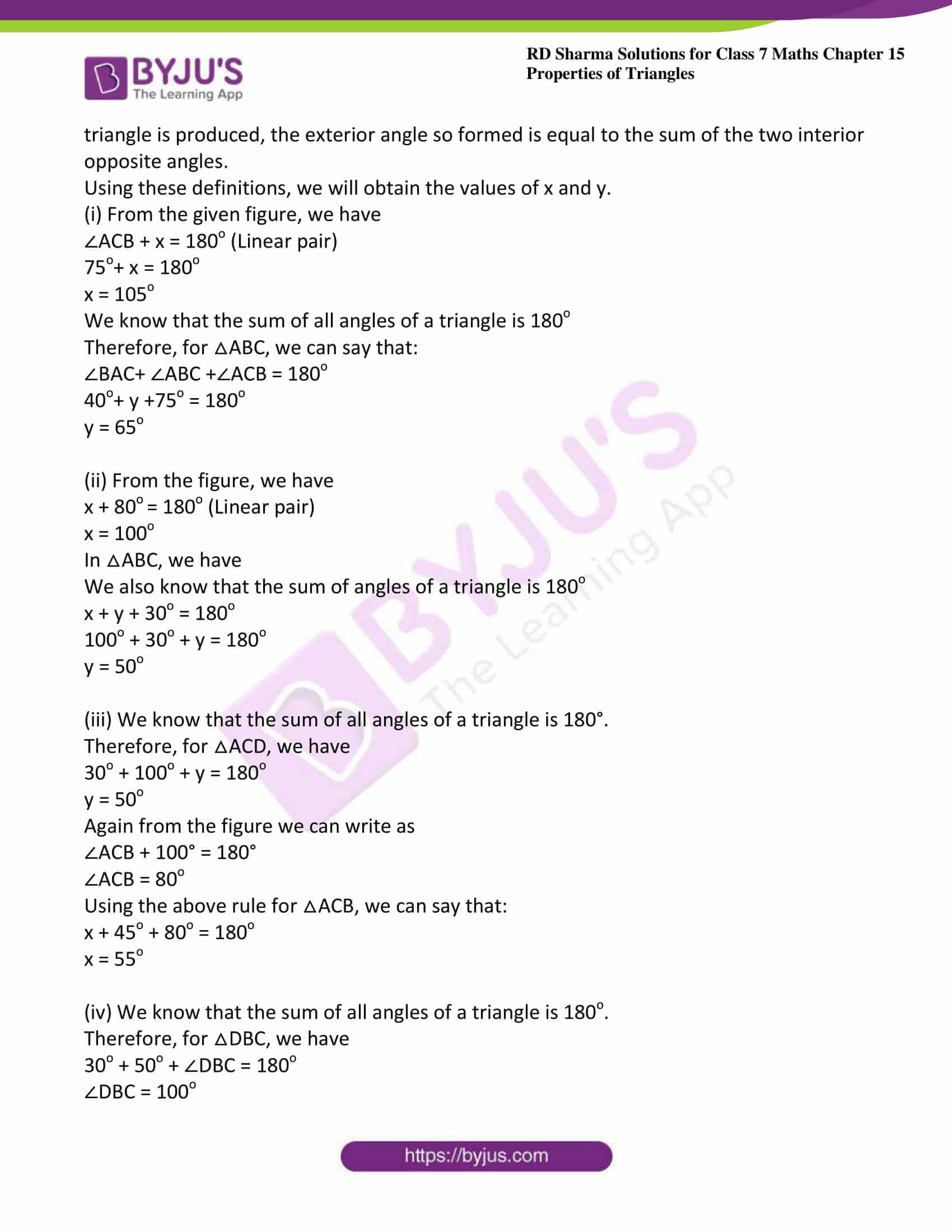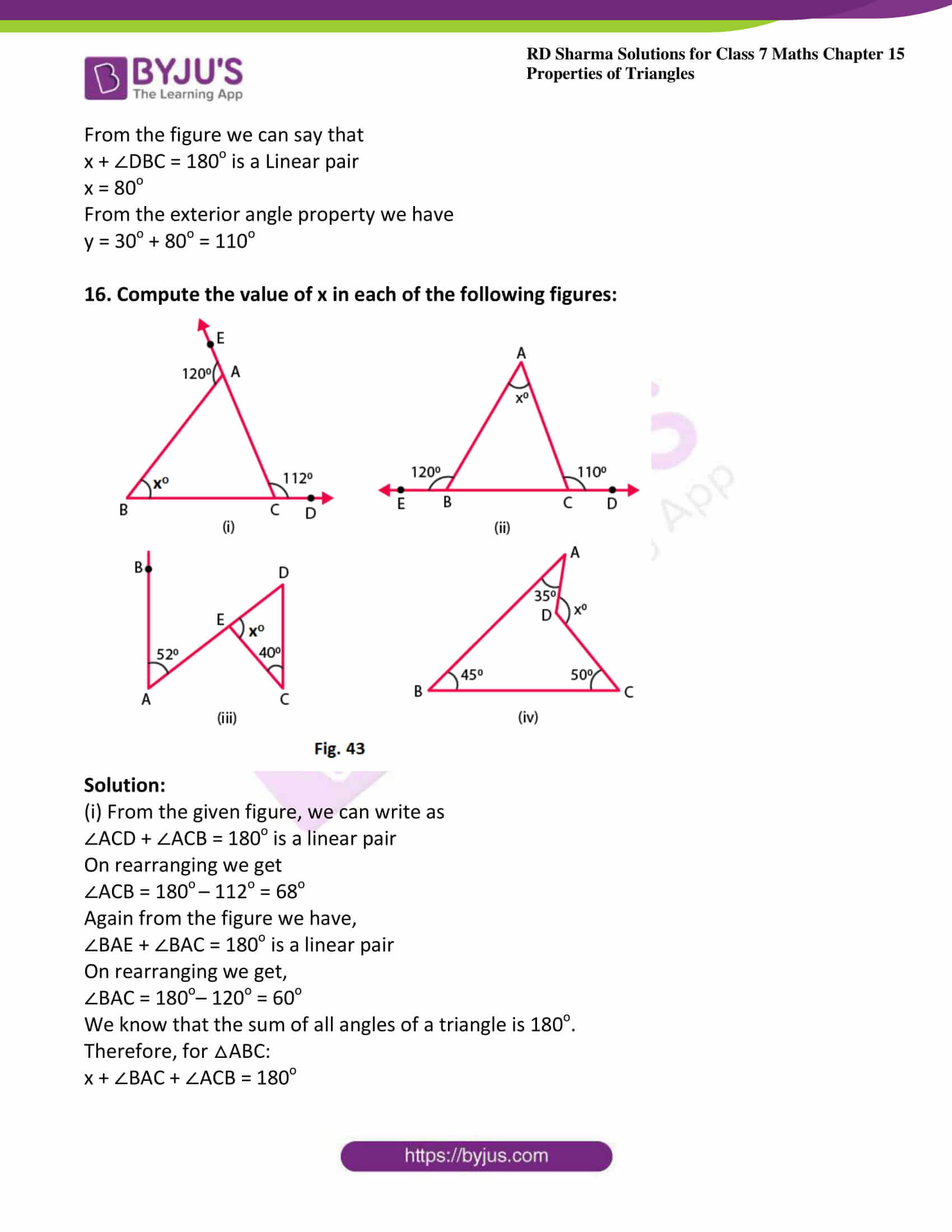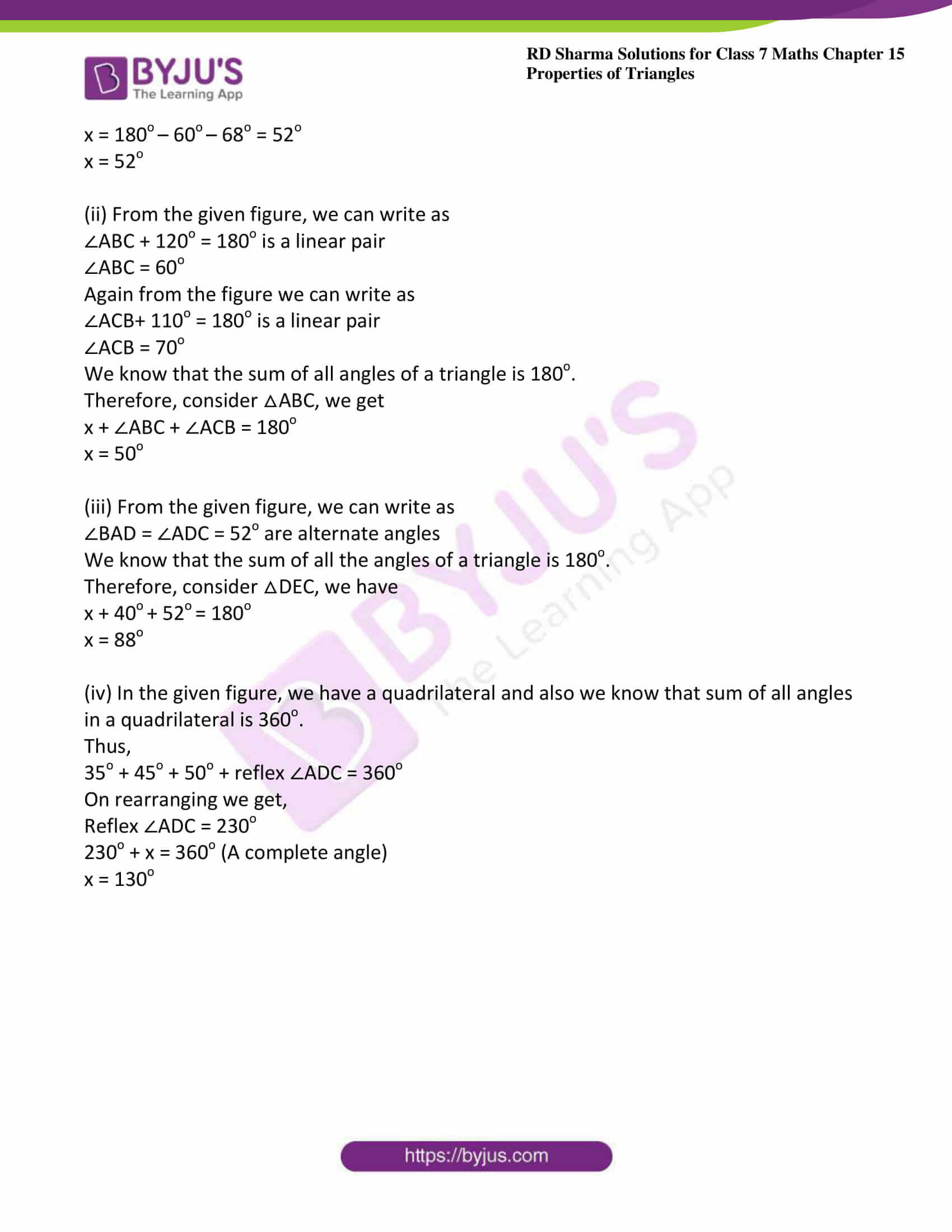### Access answers to Maths RD Sharma Solutions For Class 7 Chapter 15 – Properties of Triangles Exercise 15.3

Exercise 15.3 Page No: 15.19

1. In Fig. 35, ∠CBX is an exterior angle of ∆ABC at B. Name

(ii) The interior opposite angles to exterior ∠CBX

Also, name the interior opposite angles to an exterior angle at A.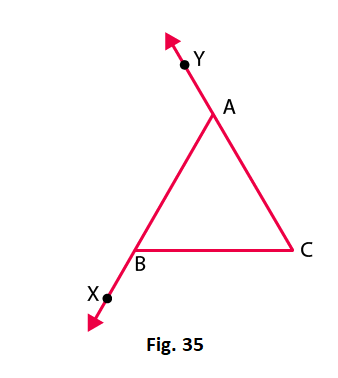Solution:

(i) The interior adjacent angle is ∠ABC

(ii) The interior opposite angles to exterior ∠CBX is ∠BAC and ∠ACB

Also the interior angles opposite to exterior are ∠ABC and ∠ACB

2. In the fig. 36, two of the angles are indicated. What are the measures of ∠ACX and ∠ACB?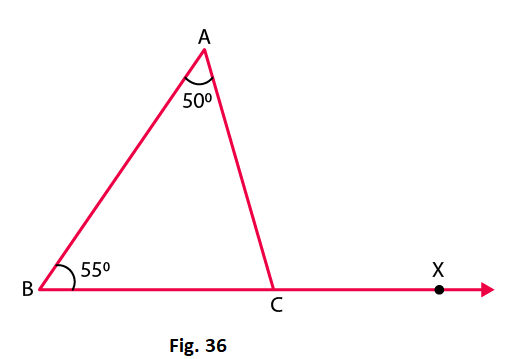Solution:

Given that in △ABC, ∠A = 50o and ∠B = 55o

We know that the sum of angles in a triangle is 180o

Therefore we have

∠A + ∠B + ∠C = 180o

50o+ 55o+ ∠C = 180o

∠C = 75o

∠ACB = 75o

∠ACX = 180o− ∠ACB = 180o − 75o = 105o

3. In a triangle, an exterior angle at a vertex is 95o and its one of the interior opposite angles is 55o. Find all the angles of the triangle.

Solution: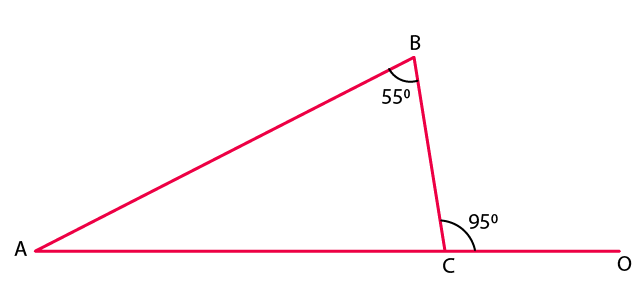We know that the sum of interior opposite angles is equal to the exterior angle.

Hence, for the given triangle, we can say that:

∠ABC+ ∠BAC = ∠BCO

55o + ∠BAC = 95o

∠BAC= 95o– 55o

∠BAC = 40o

We also know that the sum of all angles of a triangle is 180o.

Hence, for the given △ABC, we can say that:

∠ABC + ∠BAC + ∠BCA = 180o

55o + 40o + ∠BCA = 180o

∠BCA = 180o –95o

∠BCA = 85o

4. One of the exterior angles of a triangle is 80o, and the interior opposite angles are equal to each other. What is the measure of each of these two angles?

Solution:

Let us assume that A and B are the two interior opposite angles.

We know that ∠A is equal to ∠B.

We also know that the sum of interior opposite angles is equal to the exterior angle.

Therefore from the figure we have,

∠A + ∠B = 80o

∠A +∠A = 80o (because ∠A = ∠B)

2∠A = 80o

∠A = 80/2 =40o

∠A= ∠B = 40o

Thus, each of the required angles is of 40o.

5. The exterior angles, obtained on producing the base of a triangle both ways are 104o and 136o. Find all the angles of the triangle.

Solution: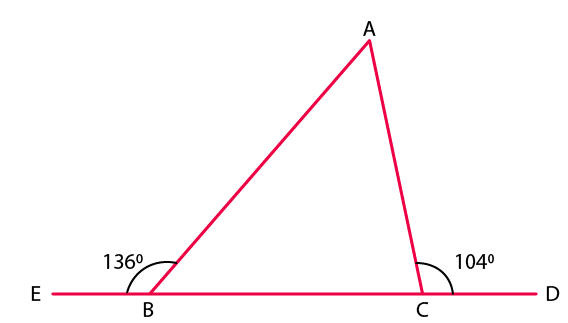In the given figure, ∠ABE and ∠ABC form a linear pair.

∠ABE + ∠ABC =180o

∠ABC = 180o– 136o

∠ABC = 44o

We can also see that ∠ACD and ∠ACB form a linear pair.

∠ACD + ∠ACB = 180o

∠ACB = 180o– 104o

∠ACB = 76o

We know that the sum of interior opposite angles is equal to the exterior angle.

Therefore, we can write as

∠BAC + ∠ABC = 104o

∠BAC = 104o – 44o = 60o

Thus,

∠ACE = 76o and ∠BAC = 60o

6. In Fig. 37, the sides BC, CA and BA of a △ABC have been produced to D, E and F respectively. If ∠ACD = 105o and ∠EAF = 45o; find all the angles of the △ABC.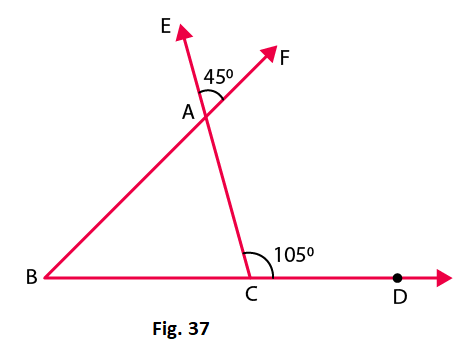Solution:

In a △ABC, ∠BAC and ∠EAF are vertically opposite angles.

Hence, we can write as

∠BAC = ∠EAF = 45o

Considering the exterior angle property, we have

∠BAC + ∠ABC = ∠ACD = 105o

On rearranging we get

∠ABC = 105o– 45o = 60o

We know that the sum of angles in a triangle is 180o

∠ABC + ∠ACB +∠BAC = 180°

∠ACB = 75o

Therefore, the angles are 45o, 60o and 75o.

7. In Fig. 38, AC perpendicular to CE and C ∠A: ∠B: ∠C= 3: 2: 1. Find the value of ∠ECD.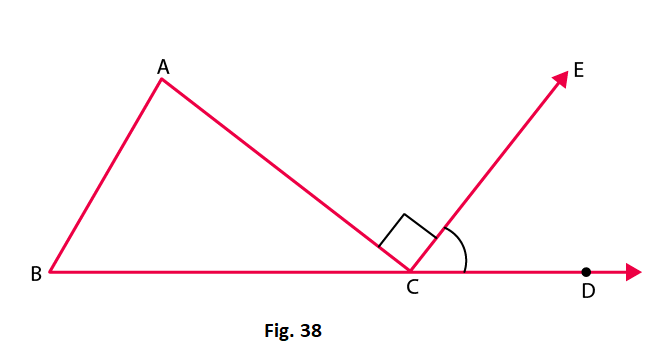Solution:

In the given triangle, the angles are in the ratio 3: 2: 1.

Let the angles of the triangle be 3x, 2x and x.

We know that sum of angles in a triangle is 180o

3x + 2x + x = 180o

6x = 180o

x = 30o

Also, ∠ACB + ∠ACE + ∠ECD = 180o

x + 90o + ∠ECD = 180o (∠ACE = 90o)

We know that x = 30o

Therefore

∠ECD = 60o

8. A student when asked to measure two exterior angles of △ABC observed that the exterior angles at A and B are of 103o and 74o respectively. Is this possible? Why or why not?

Solution:

We know that sum of internal and external angle is equal to 180o

Internal angle at A + External angle at A = 180o

Internal angle at A + 103o =180o

Internal angle at A = 77o

Internal angle at B + External angle at B = 180o

Internal angle at B + 74o = 180o

Internal angle at B = 106o

Sum of internal angles at A and B = 77o + 106o = 183o

It means that the sum of internal angles at A and B is greater than 180o, which cannot be possible.

9. In Fig.39, AD and CF are respectively perpendiculars to sides BC and AB of △ABC. If ∠FCD = 50o, find ∠BAD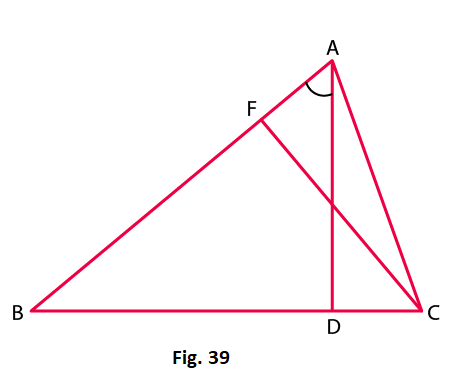Solution:

We know that the sum of all angles of a triangle is 180o

Therefore, for the given △FCB, we have

∠FCB + ∠CBF + ∠BFC = 180o

50o + ∠CBF + 90o = 180o

∠CBF = 180o – 50o – 90o = 40o

Using the above steps for △ABD, we can say that:

∠ABD + ∠BDA + ∠BAD = 180o

∠BAD = 180o – 90o – 40o = 50o

10. In Fig.40, measures of some angles are indicated. Find the value of x.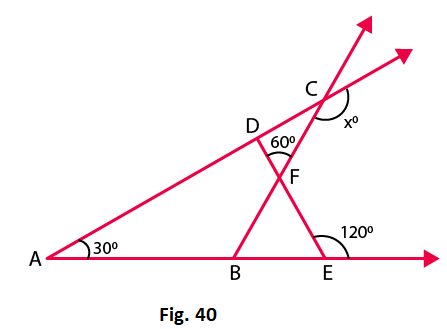Solution:

We know that the sum of the angles of a triangle is 180o

From the figure we have,

∠AED + 120o = 180o (Linear pair)

∠AED = 180o – 120o = 60o

We know that the sum of all angles of a triangle is 180o.

∠ADE + ∠AED + ∠DAE = 180o

∠ADE = 180o – 60o – 30o = 90o

From the given figure, we have

∠FDC + 90o = 180o (Linear pair)

∠FDC = 180o – 90o = 90o

Using the same steps for △CDF, we get

∠CDF + ∠DCF + ∠DFC = 180o

90o + ∠DCF + 60o = 180o

∠DCF = 180o – 60o – 90o = 30o

Again from the figure we have

∠DCF + x = 180o (Linear pair)

30o + x = 180o

x = 180o – 30o = 150o

11. In Fig. 41, ABC is a right triangle right angled at A. D lies on BA produced and DE perpendicular to BC intersecting AC at F. If ∠AFE = 130o, find

(i) ∠BDE

(ii) ∠BCA

(iii) ∠ABC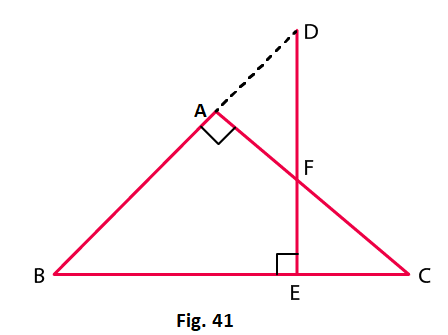Solution:

(i) Here,

∠BAF + ∠FAD = 180o (Linear pair)

∠FAD = 180o – ∠BAF = 180o – 90o = 90o

Also from the figure,

∠ADF = 130o − 90o = 40o

∠BDE = 40o

(ii) We know that the sum of all the angles of a triangle is 180o.

Therefore, for △BDE, we have

∠BDE + ∠BED + ∠DBE = 180o

∠DBE = 180o – ∠BDE – ∠BED

∠DBE = 180o – 40o – 90o = 50o …. Equation (i)

Again from the figure we have,

∠FAD = ∠ABC + ∠ACB (Exterior angle property)

90o = 50o + ∠ACB

∠ACB = 90o – 50o = 40o

(iii) From equation we have

∠ABC = ∠DBE = 50o

12. ABC is a triangle in which ∠B = ∠C and ray AX bisects the exterior angle DAC. If ∠DAX = 70o. Find ∠ACB.

Solution: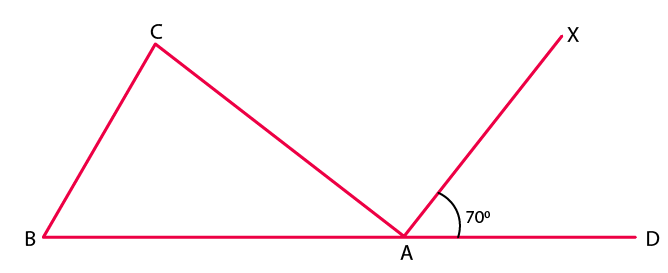Given that ABC is a triangle in which ∠B = ∠C

Also given that AX bisects the exterior angle DAC

∠CAX = ∠DAX (AX bisects ∠CAD)

∠CAX =70o [given]

∠CAX +∠DAX + ∠CAB =180o

70o+ 70o + ∠CAB =180o

∠CAB =180o –140o

∠CAB =40o

∠ACB + ∠CBA + ∠CAB = 180o (Sum of the angles of ∆ABC)

∠ACB + ∠ACB+ 40o = 180o (∠C = ∠B)

2∠ACB = 180o – 40o

∠ACB = 140/2

∠ACB = 70o

13. The side BC of △ABC is produced to a point D. The bisector of ∠A meets side BC in L. If ∠ABC= 30o and ∠ACD = 115o, find ∠ALC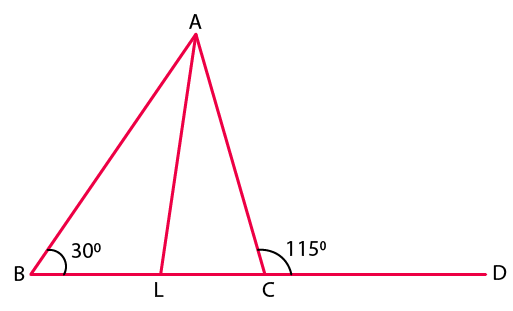Solution:

Given that ∠ABC= 30o and ∠ACD = 115o

From the figure, we have

∠ACD and ∠ACL make a linear pair.

∠ACD+ ∠ACB = 180o

115o + ∠ACB =180o

∠ACB = 180o – 115o

∠ACB = 65o

We know that the sum of all angles of a triangle is 180o.

Therefore, for△ ABC, we have

∠ABC + ∠BAC + ∠ACB = 180o

30o + ∠BAC + 65o = 180o

∠BAC = 85o

∠LAC = ∠BAC/2 = 85/2

Using the same steps for △ALC, we get

∠ALC + ∠LAC + ∠ACL = 180o

∠ALC + 85/2 + 65o = 180o

We know that ∠ACL = ∠ACB

∠ALC = 180o – 85/2 – 65o

∠ALC = 72 ½o

14. D is a point on the side BC of △ABC. A line PDQ through D, meets side AC in P and AB produced at Q. If ∠A = 80o, ∠ABC = 60o and ∠PDC = 15o, find

(i) ∠AQD

(ii) ∠APD

Solution: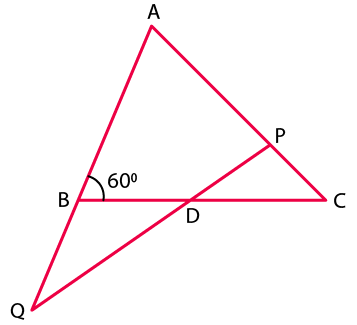From the figure we have

∠ABD and ∠QBD form a linear pair.

∠ABC + ∠QBC =180o

60o + ∠QBC = 180o

∠QBC = 120o

∠PDC = ∠BDQ (Vertically opposite angles)

∠BDQ = 15o

(i) In △QBD:

∠QBD + ∠QDB + ∠BQD = 180o (Sum of angles of △QBD)

120o+ 15o + ∠BQD = 180o

∠BQD = 180o – 135o

∠BQD = 45o

∠AQD = ∠BQD = 45o

(ii) In △AQP:

∠QAP + ∠AQP + ∠APQ = 180o (Sum of angles of △AQP)

80o + 45o + ∠APQ = 180o

∠APQ = 55o

∠APD = ∠APQ = 55o

15. Explain the concept of interior and exterior angles and in each of the figures given below. Find x and y (Fig. 42)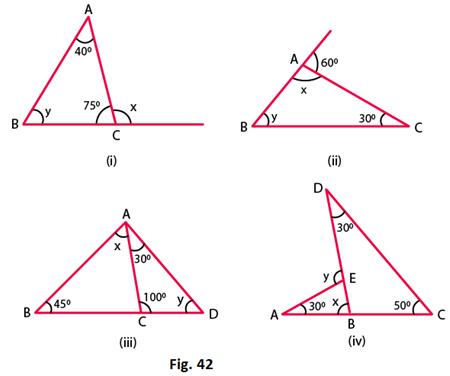Solution:

The interior angles of a triangle are the three angle elements inside the triangle.

The exterior angles are formed by extending the sides of a triangle, and if the side of a triangle is produced, the exterior angle so formed is equal to the sum of the two interior opposite angles.

Using these definitions, we will obtain the values of x and y.

(i) From the given figure, we have

∠ACB + x = 180o (Linear pair)

75o+ x = 180o

x = 105o

We know that the sum of all angles of a triangle is 180o

Therefore, for △ABC, we can say that:

∠BAC+ ∠ABC +∠ACB = 180o

40o+ y +75o = 180o

y = 65o

(ii) From the figure, we have

x + 80o = 180o (Linear pair)

x = 100o

In △ABC, we have

We also know that the sum of angles of a triangle is 180o

x + y + 30o = 180o

100o + 30o + y = 180o

y = 50o

(iii) We know that the sum of all angles of a triangle is 180°.

Therefore, for △ACD, we have

30o + 100o + y = 180o

y = 50o

Again from the figure we can write as

∠ACB + 100° = 180°

∠ACB = 80o

Using the above rule for △ACB, we can say that:

x + 45o + 80o = 180o

x = 55o

(iv) We know that the sum of all angles of a triangle is 180o.

Therefore, for △DBC, we have

30o + 50o + ∠DBC = 180o

∠DBC = 100o

From the figure we can say that

x + ∠DBC = 180o is a Linear pair

x = 80o

From the exterior angle property we have

y = 30o + 80o = 110o

16. Compute the value of x in each of the following figures: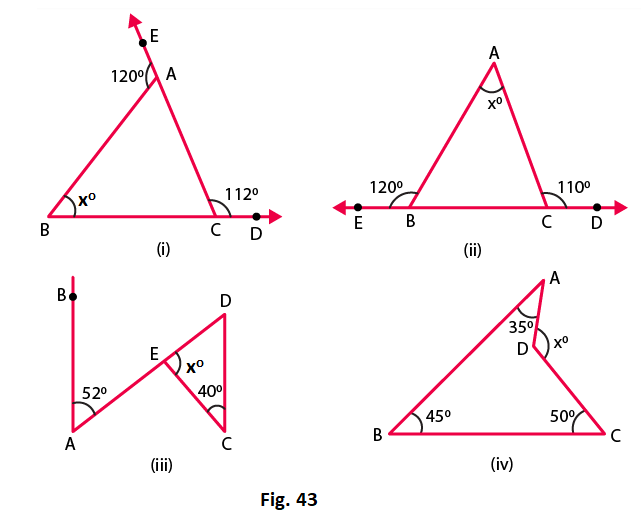Solution:

(i) From the given figure, we can write as

∠ACD + ∠ACB = 180o is a linear pair

On rearranging we get

∠ACB = 180o – 112o = 68o

Again from the figure we have,

∠BAE + ∠BAC = 180o is a linear pair

On rearranging we get,

∠BAC = 180o– 120o = 60o

We know that the sum of all angles of a triangle is 180o.

Therefore, for △ABC:

x + ∠BAC + ∠ACB = 180o

x = 180o – 60o – 68o = 52o

x = 52o

(ii) From the given figure, we can write as

∠ABC + 120o = 180o is a linear pair

∠ABC = 60o

Again from the figure we can write as

∠ACB+ 110o = 180o is a linear pair

∠ACB = 70o

We know that the sum of all angles of a triangle is 180o.

Therefore, consider △ABC, we get

x + ∠ABC + ∠ACB = 180o

x = 50o

(iii) From the given figure, we can write as

We know that the sum of all the angles of a triangle is 180o.

Therefore, consider △DEC, we have

x + 40o + 52o = 180o

x = 88o

(iv) In the given figure, we have a quadrilateral and also we know that sum of all angles in a quadrilateral is 360o.

Thus,

35o + 45o + 50o + reflex ∠ADC = 360o

On rearranging we get,